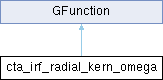GammaLib  1.7.0.dev

Kernel for radial model azimuth angle IRF integration. More...

#include <GCTAResponse_helpers.hpp>## Public Member Functions

cta_irf_radial_kern_omega (const GCTAResponseIrf *rsp, const double &zenith, const double &azimuth, const GEnergy &srcEng, const GEnergy &obsEng, const double &zeta, const double &lambda, const double &omega0, const double &rho, const double &cos_psf, const double &sin_psf, const double &cos_ph, const double &sin_ph)

double eval (const double &omega)
Kernel for radial model azimuth angle IRF integration. More...Public Member Functions inherited from GFunction
GFunction (void)
Void constructor. More...

GFunction (const GFunction &function)
Copy constructor. More...

virtual ~GFunction (void)
Destructor. More...

GFunctionoperator= (const GFunction &function)
Assignment operator. More...

## Protected Attributes

const GCTAResponseIrfm_rsp
CTA response. More...

double m_zenith
Zenith angle. More...

double m_azimuth
Azimuth angle. More...

GEnergy m_srcEng
True photon energy. More...

GEnergy m_obsEng
Measured event energy. More...

double m_zeta
Distance model centre - measured photon. More...

double m_lambda
Distance model centre - pointing. More...

double m_omega0
Azimuth of pointing in model system. More...

double m_rho
... More...

double m_cos_psf
Cosine term for PSF offset angle computation. More...

double m_sin_psf
Sine term for PSF offset angle computation. More...

double m_cos_ph
Cosine term for photon offset angle computation. More...

double m_sin_ph
Sine term for photon offset angle computation. More...Protected Member Functions inherited from GFunction
void init_members (void)
Initialise class members. More...

void copy_members (const GFunction &function)
Copy class members. More...

void free_members (void)
Delete class members. More...

## Detailed Description

Kernel for radial model azimuth angle IRF integration.

This class implements the computation of the IRF in the reference frame of the radial source model. It computes

$IRF(\rho, \omega)$

where

• $$\rho$$ is the distance from the model centre, and
• $$\omega$$ is the azimuth angle is the position angle with respect to the connecting line between the model centre and the observed photon arrival direction.

Definition at line 246 of file GCTAResponse_helpers.hpp.

## Constructor & Destructor Documentation

 cta_irf_radial_kern_omega::cta_irf_radial_kern_omega ( const GCTAResponseIrf * rsp, const double & zenith, const double & azimuth, const GEnergy & srcEng, const GEnergy & obsEng, const double & zeta, const double & lambda, const double & omega0, const double & rho, const double & cos_psf, const double & sin_psf, const double & cos_ph, const double & sin_ph )
inline

Definition at line 248 of file GCTAResponse_helpers.hpp.

## Member Function Documentation

 double cta_irf_radial_kern_omega::eval ( const double & omega )
virtual

Kernel for radial model azimuth angle IRF integration.

Parameters

Computes the kernel

$IRF(\rho,\omega)$

for the azimuth angle integration of radial models.

From the model coordinates $$(\rho,\omega)$$, the method computes the angle between the true ( $$\vec{p}$$) and observed ( $$\vec{p'}$$) photon arrival direction using

$\delta = \arccos(\cos \rho \cos \zeta + \sin \rho \sin \zeta \cos \omega)$

where $$\zeta$$ is the angular distance between the observed photon arrival direction $$\vec{p'}$$ and the model centre $$\vec{m}$$. This angle $$\delta$$ is used to compute the $$PSF(\delta)$$ value.

The method computes also the angle $$\theta$$ between the observed photon arrival direction $$\vec{p'}$$ and the camera pointing $$\vec{d}$$ using

$\theta = \arccos(\cos \rho \cos \lambda + \sin \rho \sin \lambda \cos \omega_0 - \omega)$

where $$\lambda$$ is the angular distance between the model centre $$\vec{m}$$ and the camera pointing direction $$\vec{d}$$. The angle $$\theta$$ is used in the computation of the IRF (no azimuthal dependence is so far implemented for the IRF computation).

Implements GFunction.

Definition at line 473 of file GCTAResponse_helpers.cpp.

## Member Data Documentation

protected

Azimuth angle.

Definition at line 278 of file GCTAResponse_helpers.hpp.

Referenced by eval().

protected

Cosine term for photon offset angle computation.

Definition at line 287 of file GCTAResponse_helpers.hpp.

Referenced by eval().

protected

Cosine term for PSF offset angle computation.

Definition at line 285 of file GCTAResponse_helpers.hpp.

Referenced by eval().

protected

Distance model centre - pointing.

Definition at line 282 of file GCTAResponse_helpers.hpp.

protected

Measured event energy.

Definition at line 280 of file GCTAResponse_helpers.hpp.

Referenced by eval().

protected

Azimuth of pointing in model system.

Definition at line 283 of file GCTAResponse_helpers.hpp.

Referenced by eval().

protected

...

Definition at line 284 of file GCTAResponse_helpers.hpp.

protected

CTA response.

Definition at line 276 of file GCTAResponse_helpers.hpp.

Referenced by eval().

protected

Sine term for photon offset angle computation.

Definition at line 288 of file GCTAResponse_helpers.hpp.

Referenced by eval().

protected

Sine term for PSF offset angle computation.

Definition at line 286 of file GCTAResponse_helpers.hpp.

Referenced by eval().

protected

True photon energy.

Definition at line 279 of file GCTAResponse_helpers.hpp.

Referenced by eval().

protected

Zenith angle.

Definition at line 277 of file GCTAResponse_helpers.hpp.

Referenced by eval().